|

# 安华卫浴新中式定制空间评测-祥瑞千年

祥云是最具代表性的中国文化符号之一，在中国古代的传说中是神仙所驾的彩云，被认为是祥瑞之兆，而这一概念跨越千年直到现在。今天我们要测评的这款安华卫浴新中式定制空间就融入了祥云这一古典元素，并结合当前的时代背景进行了重新演绎。现在，就随小编一起来感受这独特的文化韵味吧！

安华卫浴新中式定制空间外观测评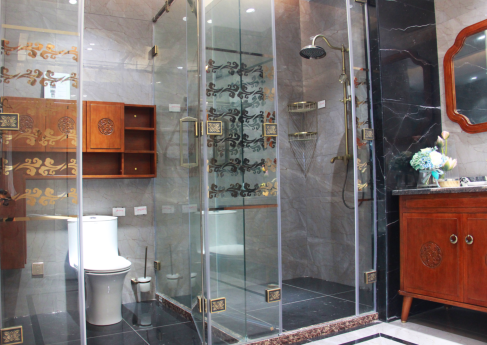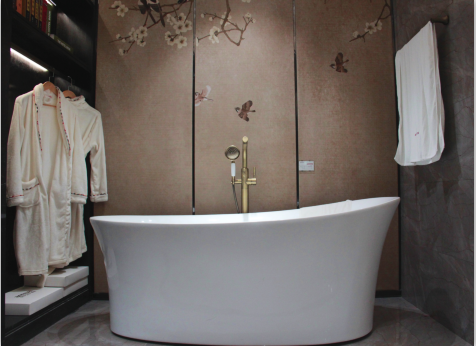整体外观。安华卫浴新中式定制空间的外观给人的第一印象深沉内敛，很是端庄典雅。看似沉闷的颜色因为有了细节的点缀，变得灵动起来。而且，整个空间内柜子、门板等的尺寸和数量都可以根据家庭的实际需求进行定制，适用于不同户型。细节点缀。为了达到整体视觉的统一，这款新中式整体空间从雕花、拉环、花纹等细节点缀方面均运用了古典元素。如淋浴房玻璃上大面积的祥云，金属配件的红古铜雕花拉丝、收纳柜上的古典雕花设计以及圆环拉手，无一不散发着浓郁的古典文化气息。立体设计。柜体的上的板面凸起处看似简单的设计实则藏着“小心机”，它采用的是传统老式柜子的设计，可以让整个柜子看起来更加立体有质感，小编看到她的第一眼，错觉里面会不会藏着外婆给我准备的果品。

安华卫浴新中式定制空间触感测评安华卫浴新中式定制空间的所有五金配件均采用青古铜，色泽深沉，手感粗粝厚实，一则方便调控，二则既符合现代家庭讲究豪华、脱俗的风格，又符合大众追求至高享受的氛围。手持花洒则在青古铜的基础上镶嵌了玉石，手感相比金属物更加温润柔和。

安华卫浴新中式定制空间细节测评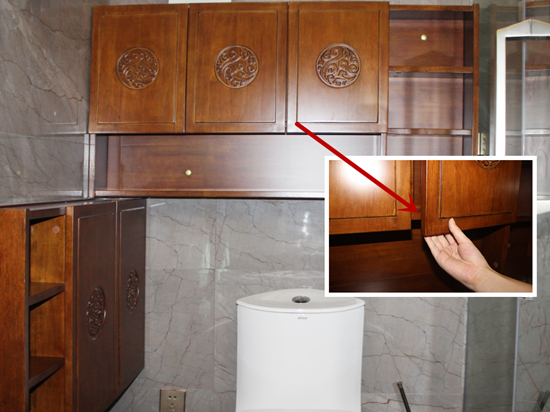坐便柜采用无拉手设计，这样一方面在视觉上整体更加简洁大气，同时也避免了拉手太高“欺负”小个子。

安华卫浴新中式定制空间功能测评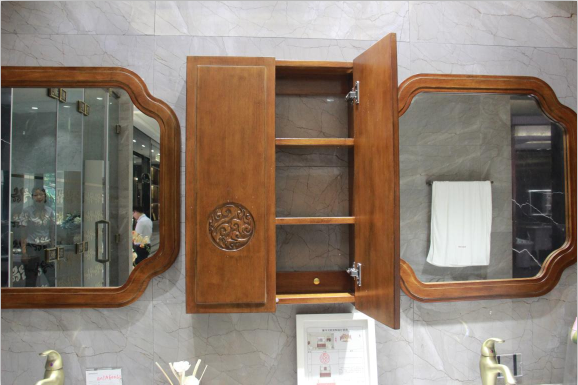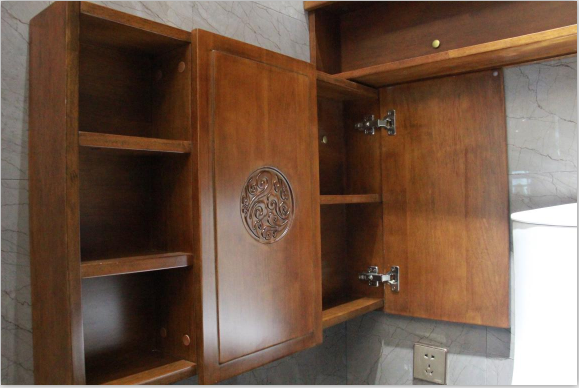收纳功能。卫浴间的收纳问题常常让人很头疼，一是物品数量多；二是物品种类多；三是物品干湿不一。要解决这三大难题，就要求卫浴间不仅收纳空间大，而且还能有不同的功能分区，让不同的物件适得其所。

安华卫浴新中式空间的储物功能绝对让人惊喜，其隔层储物柜+抽屉+展示台，让所有东西都能被安防在合适的空间，收藏与展示相结合，告别凌乱的浴室生活，每天都有好心情。镜面清晰。“爱美之心，人皆有之”，而对于爱美的人来说，镜子绝对是一个重要的存在，镜面成像清晰，能更好地帮助人们整理仪表，自信一整天。在对安华卫浴浴室镜进行清晰度测评时，小编对着镜子点火，可以看到火焰完整、清晰，无变形。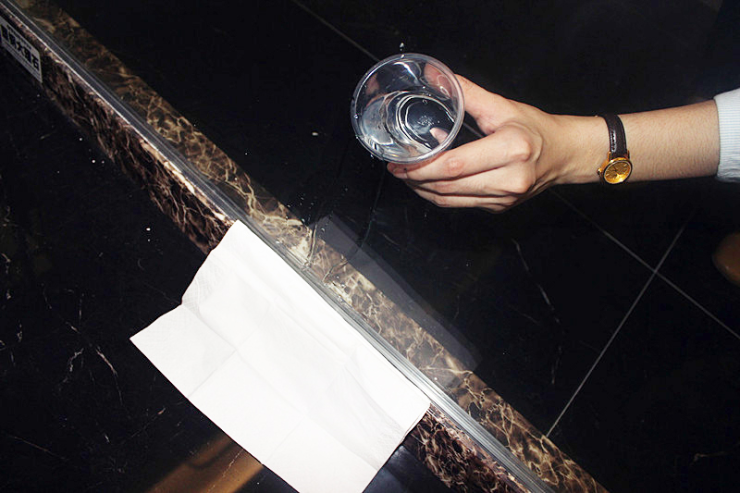干湿分离。传统的浴室设备洗完澡后到处充满水汽，长期处于这种潮湿的环境不仅影响卫浴空间的洁净，也会造成室内空气污染，因此干湿分离成为了备受人们关心的问题，也被纳入现在卫浴设计的重要部分。

安华卫浴淋浴房的玻璃门底部设有挡水胶条和导水件，测评人员在玻璃门的一边铺上干燥的纸巾，再往另一边的接口处倒水，纸巾依旧干燥如初，干湿分离的效果显而易见。表面抗污。除了梳妆台，女孩子们也会在卫浴间化妆，难免会将彩妆印上镜子或者洗手台上，如果不容易清理干净，则会影响使用。为了测试安华卫浴浴室镜的抗污力，小编咬咬牙贡献出自己的宝贝唇膏，在镜子上写了“annwa”字样，过一会儿再用纸巾擦拭，很容易就能擦除干净，明亮如新。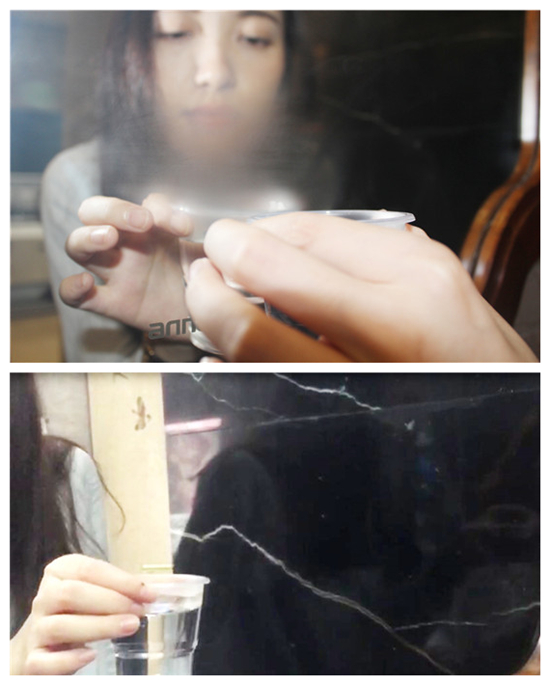防雾气。很多用户家庭的浴室镜会因为水汽氤氲被蒙上一层雾，影响使用。虽然安华卫浴的浴室空间在干湿分离方面表现很好，但是由于雾气遮掩也是用户使用浴室镜的痛点之一，我们还是对这一性能进行了测评。

测评人员将一杯热水靠近镜子，然后对着镜子吹起，使雾气蒙上镜面，移开水杯，雾气很快就消失了。

安华卫浴新中式定制空间可定制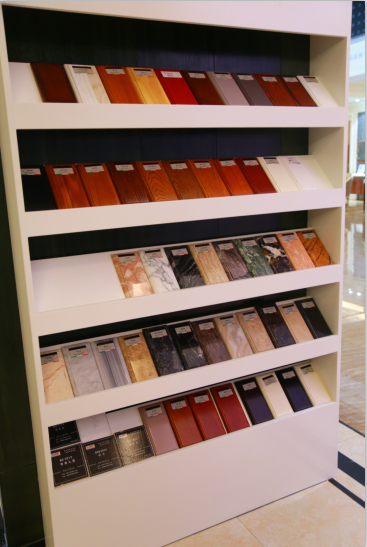安华卫浴新中式定制空间除了尺寸和柜体的数量可定制外，洗手台的石材和柜体的的木材也有各种材质可选，满足不同的用户需求，让对传统文化感兴趣的人们都能接受古典文化的浸润。

小编总结：经测评，安华卫浴新中式定制空间不管是在文化传承、外观造型、细节点缀还是在材质性能等方面都有良好的表现，尤其是她将古典元素在有限的空间里的完美演绎，热爱传统文化的人或许能从其中感受到无限的文化韵味与高雅气质。

在整体卫浴空间的定制上，安华卫浴为你提供多样化的选择，不管是浴室柜、淋浴房还是浴缸，你都可以根据自己的喜好以及空间需求，挑选卫浴产品的颜色、尺寸、结构、材质、样式、风格等，定制独一无二的卫浴空间，让你的卫浴生活更和谐，更有质感，也更加舒适。

`声明：本文由入驻焦点开放平台的作者撰写，除焦点官方账号外，观点仅代表作者本人，不代表焦点立场错误信息举报电话： 400-099-0099，邮箱：jubao@vip.sohu.com，或点此进行意见反馈，或点此进行举报投诉。`A B C D E F G H J K L M N P Q R S T W X Y Z
A - B - C - D - E
• A
• 鞍山
• 安庆
• 安阳
• 安顺
• 安康
• 澳门
• B
• 北京
• 保定
• 包头
• 巴彦淖尔
• 本溪
• 蚌埠
• 亳州
• 滨州
• 北海
• 百色
• 巴中
• 毕节
• 保山
• 宝鸡
• 白银
• 巴州
• C
• 承德
• 沧州
• 长治
• 赤峰
• 朝阳
• 长春
• 常州
• 滁州
• 池州
• 长沙
• 常德
• 郴州
• 潮州
• 崇左
• 重庆
• 成都
• 楚雄
• 昌都
• 慈溪
• 常熟
• D
• 大同
• 大连
• 丹东
• 大庆
• 东营
• 德州
• 东莞
• 德阳
• 达州
• 大理
• 德宏
• 定西
• 儋州
• 东平
• E
• 鄂尔多斯
• 鄂州
• 恩施
F - G - H - I - J
• F
• 抚顺
• 阜新
• 阜阳
• 福州
• 抚州
• 佛山
• 防城港
• G
• 赣州
• 广州
• 桂林
• 贵港
• 广元
• 广安
• 贵阳
• 固原
• H
• 邯郸
• 衡水
• 呼和浩特
• 呼伦贝尔
• 葫芦岛
• 哈尔滨
• 黑河
• 淮安
• 杭州
• 湖州
• 合肥
• 淮南
• 淮北
• 黄山
• 菏泽
• 鹤壁
• 黄石
• 黄冈
• 衡阳
• 怀化
• 惠州
• 河源
• 贺州
• 河池
• 海口
• 红河
• 汉中
• 海东
• 怀来
• I
• J
• 晋中
• 锦州
• 吉林
• 鸡西
• 佳木斯
• 嘉兴
• 金华
• 景德镇
• 九江
• 吉安
• 济南
• 济宁
• 焦作
• 荆门
• 荆州
• 江门
• 揭阳
• 金昌
• 酒泉
• 嘉峪关
K - L - M - N - P
• K
• 开封
• 昆明
• 昆山
• L
• 廊坊
• 临汾
• 辽阳
• 连云港
• 丽水
• 六安
• 龙岩
• 莱芜
• 临沂
• 聊城
• 洛阳
• 漯河
• 娄底
• 柳州
• 来宾
• 泸州
• 乐山
• 六盘水
• 丽江
• 临沧
• 拉萨
• 林芝
• 兰州
• 陇南
• M
• 牡丹江
• 马鞍山
• 茂名
• 梅州
• 绵阳
• 眉山
• N
• 南京
• 南通
• 宁波
• 南平
• 宁德
• 南昌
• 南阳
• 南宁
• 内江
• 南充
• P
• 盘锦
• 莆田
• 平顶山
• 濮阳
• 攀枝花
• 普洱
• 平凉
Q - R - S - T - W
• Q
• 秦皇岛
• 齐齐哈尔
• 衢州
• 泉州
• 青岛
• 清远
• 钦州
• 黔南
• 曲靖
• 庆阳
• R
• 日照
• 日喀则
• S
• 石家庄
• 沈阳
• 双鸭山
• 绥化
• 上海
• 苏州
• 宿迁
• 绍兴
• 宿州
• 三明
• 上饶
• 三门峡
• 商丘
• 十堰
• 随州
• 邵阳
• 韶关
• 深圳
• 汕头
• 汕尾
• 三亚
• 三沙
• 遂宁
• 山南
• 商洛
• 石嘴山
• T
• 天津
• 唐山
• 太原
• 通辽
• 铁岭
• 泰州
• 台州
• 铜陵
• 泰安
• 铜仁
• 铜川
• 天水
• 天门
• W
• 乌海
• 乌兰察布
• 无锡
• 温州
• 芜湖
• 潍坊
• 威海
• 武汉
• 梧州
• 渭南
• 武威
• 吴忠
• 乌鲁木齐
X - Y - Z
• X
• 邢台
• 徐州
• 宣城
• 厦门
• 新乡
• 许昌
• 信阳
• 襄阳
• 孝感
• 咸宁
• 湘潭
• 湘西
• 西双版纳
• 西安
• 咸阳
• 西宁
• 仙桃
• 西昌
• Y
• 运城
• 营口
• 盐城
• 扬州
• 鹰潭
• 宜春
• 烟台
• 宜昌
• 岳阳
• 益阳
• 永州
• 阳江
• 云浮
• 玉林
• 宜宾
• 雅安
• 玉溪
• 延安
• 榆林
• 银川
• Z
• 张家口
• 镇江
• 舟山
• 漳州
• 淄博
• 枣庄
• 郑州
• 周口
• 驻马店
• 株洲
• 张家界
• 珠海
• 湛江
• 肇庆
• 中山
• 自贡
• 资阳
• 遵义
• 昭通
• 张掖
• 中卫

1室1厅1厨1卫1阳台

1
2
3
4
5

0
1
2

1

1

0
1
2
3报名成功，资料已提交审核A B C D E F G H J K L M N P Q R S T W X Y Z
A - B - C - D - E
• A
• 鞍山
• 安庆
• 安阳
• 安顺
• 安康
• 澳门
• B
• 北京
• 保定
• 包头
• 巴彦淖尔
• 本溪
• 蚌埠
• 亳州
• 滨州
• 北海
• 百色
• 巴中
• 毕节
• 保山
• 宝鸡
• 白银
• 巴州
• C
• 承德
• 沧州
• 长治
• 赤峰
• 朝阳
• 长春
• 常州
• 滁州
• 池州
• 长沙
• 常德
• 郴州
• 潮州
• 崇左
• 重庆
• 成都
• 楚雄
• 昌都
• 慈溪
• 常熟
• D
• 大同
• 大连
• 丹东
• 大庆
• 东营
• 德州
• 东莞
• 德阳
• 达州
• 大理
• 德宏
• 定西
• 儋州
• 东平
• E
• 鄂尔多斯
• 鄂州
• 恩施
F - G - H - I - J
• F
• 抚顺
• 阜新
• 阜阳
• 福州
• 抚州
• 佛山
• 防城港
• G
• 赣州
• 广州
• 桂林
• 贵港
• 广元
• 广安
• 贵阳
• 固原
• H
• 邯郸
• 衡水
• 呼和浩特
• 呼伦贝尔
• 葫芦岛
• 哈尔滨
• 黑河
• 淮安
• 杭州
• 湖州
• 合肥
• 淮南
• 淮北
• 黄山
• 菏泽
• 鹤壁
• 黄石
• 黄冈
• 衡阳
• 怀化
• 惠州
• 河源
• 贺州
• 河池
• 海口
• 红河
• 汉中
• 海东
• 怀来
• I
• J
• 晋中
• 锦州
• 吉林
• 鸡西
• 佳木斯
• 嘉兴
• 金华
• 景德镇
• 九江
• 吉安
• 济南
• 济宁
• 焦作
• 荆门
• 荆州
• 江门
• 揭阳
• 金昌
• 酒泉
• 嘉峪关
K - L - M - N - P
• K
• 开封
• 昆明
• 昆山
• L
• 廊坊
• 临汾
• 辽阳
• 连云港
• 丽水
• 六安
• 龙岩
• 莱芜
• 临沂
• 聊城
• 洛阳
• 漯河
• 娄底
• 柳州
• 来宾
• 泸州
• 乐山
• 六盘水
• 丽江
• 临沧
• 拉萨
• 林芝
• 兰州
• 陇南
• M
• 牡丹江
• 马鞍山
• 茂名
• 梅州
• 绵阳
• 眉山
• N
• 南京
• 南通
• 宁波
• 南平
• 宁德
• 南昌
• 南阳
• 南宁
• 内江
• 南充
• P
• 盘锦
• 莆田
• 平顶山
• 濮阳
• 攀枝花
• 普洱
• 平凉
Q - R - S - T - W
• Q
• 秦皇岛
• 齐齐哈尔
• 衢州
• 泉州
• 青岛
• 清远
• 钦州
• 黔南
• 曲靖
• 庆阳
• R
• 日照
• 日喀则
• S
• 石家庄
• 沈阳
• 双鸭山
• 绥化
• 上海
• 苏州
• 宿迁
• 绍兴
• 宿州
• 三明
• 上饶
• 三门峡
• 商丘
• 十堰
• 随州
• 邵阳
• 韶关
• 深圳
• 汕头
• 汕尾
• 三亚
• 三沙
• 遂宁
• 山南
• 商洛
• 石嘴山
• T
• 天津
• 唐山
• 太原
• 通辽
• 铁岭
• 泰州
• 台州
• 铜陵
• 泰安
• 铜仁
• 铜川
• 天水
• 天门
• W
• 乌海
• 乌兰察布
• 无锡
• 温州
• 芜湖
• 潍坊
• 威海
• 武汉
• 梧州
• 渭南
• 武威
• 吴忠
• 乌鲁木齐
X - Y - Z
• X
• 邢台
• 徐州
• 宣城
• 厦门
• 新乡
• 许昌
• 信阳
• 襄阳
• 孝感
• 咸宁
• 湘潭
• 湘西
• 西双版纳
• 西安
• 咸阳
• 西宁
• 仙桃
• 西昌
• Y
• 运城
• 营口
• 盐城
• 扬州
• 鹰潭
• 宜春
• 烟台
• 宜昌
• 岳阳
• 益阳
• 永州
• 阳江
• 云浮
• 玉林
• 宜宾
• 雅安
• 玉溪
• 延安
• 榆林
• 银川
• Z
• 张家口
• 镇江
• 舟山
• 漳州
• 淄博
• 枣庄
• 郑州
• 周口
• 驻马店
• 株洲
• 张家界
• 珠海
• 湛江
• 肇庆
• 中山
• 自贡
• 资阳
• 遵义
• 昭通
• 张掖
• 中卫• 手机• 分享
• 设计
免费设计
• 计算器
装修计算器
• 入驻
合作入驻
• 联系
联系我们
• 置顶
返回顶部